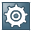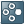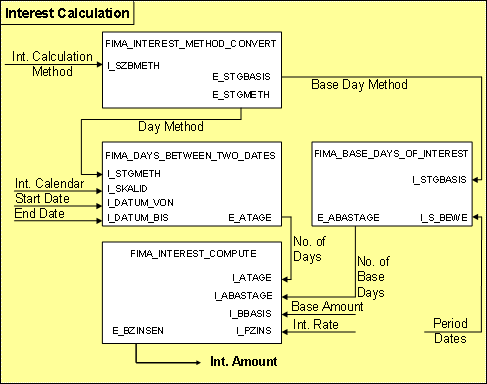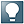##Interest Calculation### Use

Interest calculation is one of the core functions of Financial Mathematics. Interest calculation performs the calculation of interest for a calculation base amount in the calculation period by using certain Interest Calculation Methods.  Interest formulas for linear and exponential interest calculation are used.  An interest amount is the result of interest calculation.

Interest calculation is a pro rata percentage calculation in which the days for which interest is to be calculated are put in relation to the days defined for an interest year.  This is expressed in the quotient Days / Base Days.

If the quotient Days / Base Days is used as a factor in the interest rate, this is called linear interest calculation.

However, if the quotient Days / Base Days appears in the exponent, this is known as exponential interest calculation.

If broken periods exist in a calculation period for which compound interest is to be calculated linearly, this is called combined interest calculation.

Intraday interest calculation is a special case in which interest is calculated for time periods within one day.

Accordingly, interest calculation is divided into the following types:

The components of an interest formula are always the same, independent of the type of interest calculation.

Calculation base amount

Interest rate

Days

Interest calculation requires some preparation by the calling application but it can be used very flexibly. Therefore, it can deal with different types of interest and not just actual interest calculation. It can also be used to determine cash flows, that are the basis of the evaluation and the analysis of financial transactions.For example, the system calculates the interest for specified billing periods that is due for checking accounts. This includes overdraft interest as well as debit and credit interest, and other customer-specific types of interest.

The system needs the future interest payments for a loan in order to evaluate the loan. These are determined when you use the function Interest Calculation during payment flow generation.

### Integration

In individual cases interest calculation for the interest and repayment schedule occurs during cash flow calculation and the scaling of the comparison account during effective interest calculation.

The following chart shows the relationship between the Interest Calculation function and other FIMA functions:### Prerequisites

The system requires all components of an interest formula, also known as calculation bases, to execute interest calculation.

### Features

The function module FIMA_INTEREST_COMPUTE (also called the interest calculator) executes the calculation of interest in the system. The system determines the interest that is due for a calculation period, taking the interest rate and base amount into account, based on the Interest Calculation Method

The following chart depicts how the system executes interest calculation and how it calls up individual functions. The interaction between the most important parameters is also shown.First, the interest calculation method is converted into the day method and the base day method. Then the system determines the Days and the Base Days needed for the quotient of the interest formula.For more information, see Determining the Days Between Two Calendar Dates and Determining the Base Days for the Interest Calculation Method.

All the components of interest calculation are included in the function module FIMA_INTEREST_COMPUTE that supplies the resulting interest amount.

### Example

For examples of the individual types of interest calculation, see , Combined Interest Calculation and Indraday Interest Calculation.